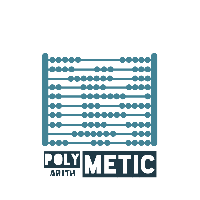Polymetic  1.1 A c++ library for polynomial and matrix arithmetic, focused on applications in Kinematics.
Class List
Here are the classes, structs, unions and interfaces with brief descriptions:
[detail level 123]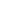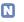anonymous_namespace{MatrixAlgorithms_impl.ipp}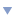anonymous_namespace{Test_Matrix_Operations.cpp}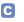MatrixTestanonymous_namespace{Test_MatrixAlgorithms_double.cpp}MatrixTestanonymous_namespace{Test_MatrixAlgorithms_polynomial_double.cpp}MatrixTestboostnumericublasPolynomial Contains the definition for the abstract base class which will be used by different multiplication algorithmsPolynomialMPZTestPolynomialMultiplicationInterface Polynomial and related classesPolynomialMultiplicationSimple Simple O(n^2) field/ring operations algorithm for univariate polynomial multiplicationPolynomialTest